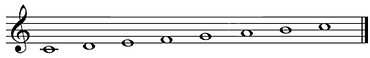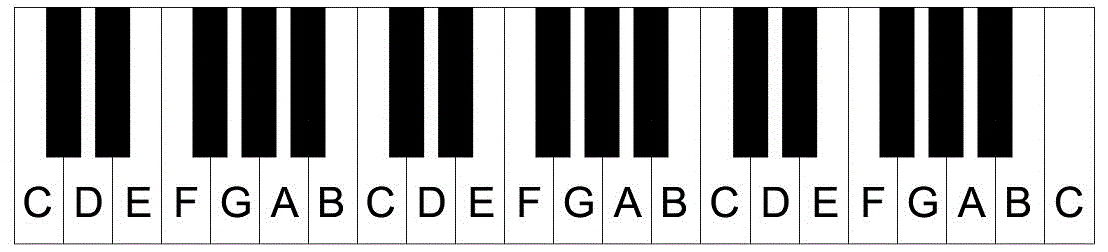## Resources

Scales

A scale is a pattern of notes. In Western music, most of our music is based around major and minor scales. The most basic scale is a C major scale. It uses only the white notes.

Every major scale follows the same pattern of intervals.

The notes in C major scale are below. Try counting how many semitones are between each note of the scale.

Hopefully the numbers you counted match up with those in the diagram above!

To clarify, C to D = 2 semitones, D to E = 2 semitones, E to F = 1 semitone (because there is no black note in between), F to G = 2 semitones, G to A = 2 semitones, A to B = 2 semitones, and B to C = 1 semitone.

An interval of 2 semitones is called a tone. We will shorten tone to T, and semitone to S. So we can think of the C major scale as following this pattern of intervals:

Interval:                                             T  T  S  T  T  T  S

Note:                                            C D E F G A B C

If we count the intervals in a G major scale, the same pattern of intervals appears:

Interval:                                              T  T  S  T  T  T   S

Note:                                            G A B C D E F# G

You do not need to memorise this pattern (T T S T T T S), but it can be really helpful to understand that every major scale is effectively the same musical pattern, just starting and ending in a different place.Intervals

An 'interval' is the gap between two notes. So different types of 'interval' have different sized gaps.

For example, the smallest possible interval is a semitone. The gap from is 1 semitone.  The gap from C - C# is also 1 semitone

A gap (or 'interval') of 2 semitones is called a tone. So The gap from F# is 1  tone.  The gap from C - D is also 1 tone